Courses

# Compatibility Condition Civil Engineering (CE) Notes | EduRev

## Civil Engineering (CE) : Compatibility Condition Civil Engineering (CE) Notes | EduRev

The document Compatibility Condition Civil Engineering (CE) Notes | EduRev is a part of Civil Engineering (CE) category.
All you need of Civil Engineering (CE) at this link: Civil Engineering (CE)

Compatibility condition
Till now we studied on ways to find the strain given a displacement field. However, there arises a need while solving boundary value problems wherein we would need to find the displacement given the strain field. This problem involves finding the 3 components of the displacement from the 6 independent components of the strain (only six components since strain is a symmetric tensor) that has been prescribed. To be able to find a continuous displacement field from the prescribed 6 components of the linearized strain, all these 6 components cannot be prescribed arbitrarily. The restrictions that these prescribed 6 components of the strain should satisfy is given by the compatibility condition. These restrictions are obtained from the requirement that the sequence of differentiation is immaterial when the first derivative of the multivariate function is continuous, i.e.,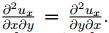. Working with index notation, the Cartesian components of the linearized strain is,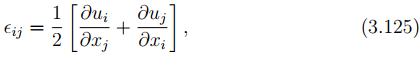where ui are the Cartesian components of the displacement vector, xi are the Cartesian coordinates of a point. Then, computing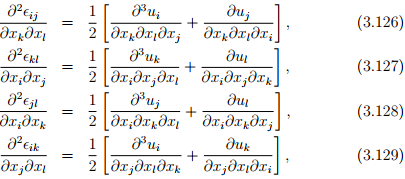we find that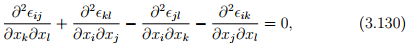since the order of differentiation is immaterial. Equation (3.130) is called Saint Venant compatibility equations. It can be also seen that equation (3.130) has 81 individual equations as each of the index - i, j, k, l - takes values 1, 2 and 3. It has been shown that of these 81 equations most are either simple identities or repetitions and only 6 are meaningful. These six equations involving the 6 independent Cartesian components of the linearized strain are: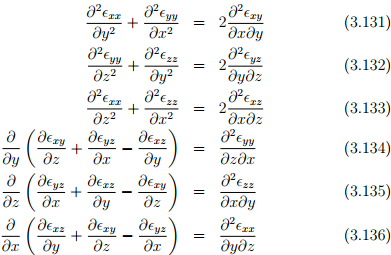Next, we show that these 6 equations are necessary conditions. For this assume ∈xy = xy and all other component of the strains are 0. For this strain it can be shown that equation (3.131) alone is violated and that no smooth displacement field would result in this state of strain. Similarly, on assuming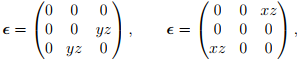we find that for each of these state of strain only one of the compatibility equations is violated; equation (3.132) for the first strain and (3.133) for the other strain. In the same fashion, on assuming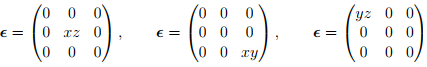we find that for each of these state of strain only one of the compatibility equations is violated; equation (3.134) for the first strain, (3.135) for the second strain and (3.136) for the last strain. Thus, we find that all the six equations are required to ensure the existence of a smooth displacement field given a strain field.

However, there are claims that the number of independent compatibility equations is only three. This is not correct for the following reasons. On differentiating the strain field four times instead of twice as done in case of compatibility condition, the six compatibility equations (3.131) through (3.136) can be shown to be equivalent to the following three equations: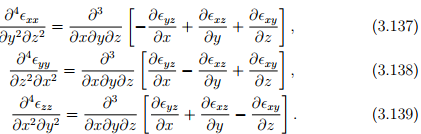This assumes existence of non-zero higher derivatives of strain field which may not be always true, as in the case of the strain field assumed above. Hence, only if higher order derivatives of strain field exist and is different from zero can one replace the 6 compatibility conditions (3.131) through (3.136) by (3.137) through (3.139).

Further, it is not easy to show that the above compatibility conditions (3.131) through (3.136) are sufficient to find a smooth displacement field given a strain field (see for example Sadd  to establish sufficiency of these conditions). In fact the sufficiency of these conditions in multiply connected8 bodies is yet to be established.

It should be mentioned that the form of the compatibility equation depends on the strain measure that is prescribed. For Cauchy-Green strain it is given in terms of the Riemannian tensor for simply connected bodies9 . However, it is still not clear what these compatibility conditions have to be for other classes of bodies when Cauchy-Green strain is used. Development of compatibility conditions for other strain measures is beyond the scope of this course.

Offer running on EduRev: Apply code STAYHOME200 to get INR 200 off on our premium plan EduRev Infinity!

,

,

,

,

,

,

,

,

,

,

,

,

,

,

,

,

,

,

,

,

,

;